### Monotone and FCS Logistic Regression Methods

The logistic regression method is another imputation method available for classification variables. In the logistic regression method, a logistic regression model is fitted for a classification variable with a set of covariates constructed from the effects. For a binary classification variable, based on the fitted regression model, a new logistic regression model is simulated from the posterior predictive distribution of the parameters and is used to impute the missing values for each variable (Rubin, 1987, pp. 167–170).

For a binary variable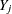with responses 1 and 2, a logistic regression model is fitted using observations with observed values for the imputed variableand its covariates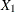,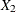, …,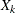: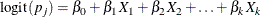where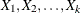are covariates for,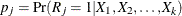,   and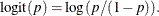The fitted model includes the regression parameter estimates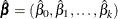and the associated covariance matrix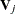.

The following steps are used to generate imputed values for a binary variablewith responses 1 and 2:

1. New parameters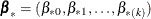are drawn from the posterior predictive distribution of the parameters.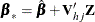where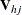is the upper triangular matrix in the Cholesky decomposition,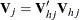, andis a vector of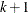independent random normal variates.

2. For an observation with missingand covariates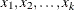, compute the expected probability that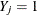: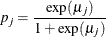where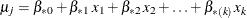.

3. Draw a random uniform variate, u, between 0 and 1. If the value of u is less than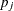, impute; otherwise impute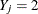.

The preceding logistic regression method can be extended to include the ordinal classification variables with more than two levels of responses. The options ORDER= and DESCENDING can be used to specify the sort order for the levels of the imputed variables.